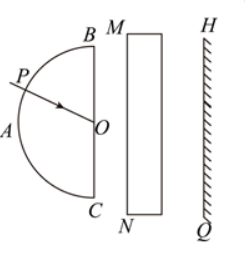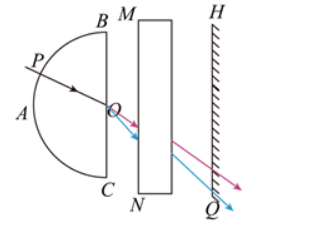$\text{A.}$ 在 $P$ 点时, 若将光屏稍向右平移, 光屏上红色与紫色光斑的间距将增大 $\text{B.}$ 在 $P$ 点时, 光屏上红色光斑在紫色光斑的下方 $\text{C.}$ 在 $P$ 点时, 若撤除长方体玻璃砖, 光屏上红色光斑将向下移动 $\text{D.}$ 从 $B$ 移到 $C$ 的过程中, 光屏上红色光斑移动的平均速率比紫色光斑的大

AC

#### 解析：C. 光线通过长方体玻璃砖后会向上发生移动, 若撤除长方体玻璃砖, 光屏上红色光斑将向下移动, 故 $C$ 正确；
$D$. 从 $B$ 移到 $C$ 的过程中, 从 $O$ 点射出的红光偏转角最小, 移动距离最小, 光屏上红色光斑移动的距离比紫色光斑移动的距 离小, 则光屏上红色光斑移动的平均速率比紫色光斑的小, 故 $D$ 错误。